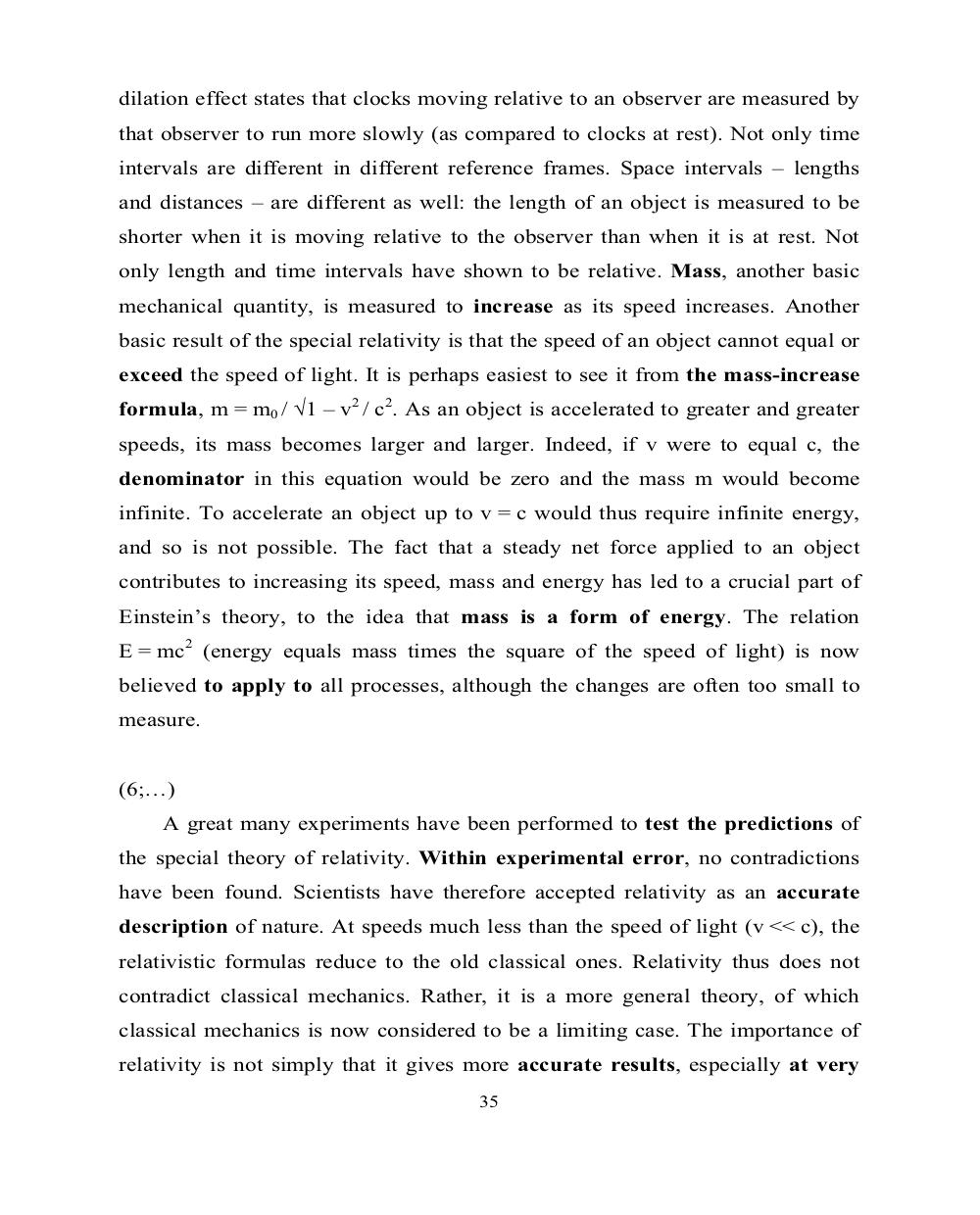# Английский язык. Ч.3 (Units 9,10). Ильичева Н.А - 35 стр.

Составители:

Рубрика:

• ## Иностранный язык

dilation effect states that clocks moving relative to an observer are measured by
that observer to run more slowly (as compared to clocks at rest). Not only time
intervals are different in different reference frames. Space intervals – lengths
and distances – are different as well: the length of an object is measured to be
shorter when it is moving relative to the observer than when it is at rest. Not
only length and time intervals have shown to be relative. Mass, another basic
mechanical quantity, is measured to increase as its speed increases. Another
basic result of the special relativity is that the speed of an object cannot equal or
exceed the speed of light. It is perhaps easiest to see it from the mass-increase
formula, m = m
0
/ ¥1 – v
2
/ c
2
. As an object is accelerated to greater and greater
speeds, its mass becomes larger and larger. Indeed, if v were to equal c, the
denominator in this equation would be zero and the mass m would become
infinite. To accelerate an object up to v = c would thus require infinite energy,
and so is not possible. The fact that a steady net force applied to an object
contributes to increasing its speed, mass and energy has led to a crucial part of
Einstein’s theory, to the idea that mass is a form of energy. The relation
E = mc
2
(energy equals mass times the square of the speed of light) is now
believed to apply to all processes, although the changes are often too small to
measure.
(6;…)
A great many experiments have been performed to test the predictions of
the special theory of relativity. Within experimental error, no contradictions
have been found. Scientists have therefore accepted relativity as an accurate
description of nature. At speeds much less than the speed of light (v << c), the
relativistic formulas reduce to the old classical ones. Relativity thus does not
contradict classical mechanics. Rather, it is a more general theory, of which
classical mechanics is now considered to be a limiting case. The importance of
relativity is not simply that it gives more accurate results, especially at very
35dilation effect states that clocks moving relative to an observer are measured by
that observer to run more slowly (as compared to clocks at rest). Not only time
intervals are different in different reference frames. Space intervals – lengths
and distances – are different as well: the length of an object is measured to be
shorter when it is moving relative to the observer than when it is at rest. Not
only length and time intervals have shown to be relative. Mass, another basic
mechanical quantity, is measured to increase as its speed increases. Another
basic result of the special relativity is that the speed of an object cannot equal or
exceed the speed of light. It is perhaps easiest to see it from the mass-increase
formula, m = m0 / �1 – v2 / c2. As an object is accelerated to greater and greater
speeds, its mass becomes larger and larger. Indeed, if v were to equal c, the
denominator in this equation would be zero and the mass m would become
infinite. To accelerate an object up to v = c would thus require infinite energy,
and so is not possible. The fact that a steady net force applied to an object
contributes to increasing its speed, mass and energy has led to a crucial part of
Einstein’s theory, to the idea that mass is a form of energy. The relation
E = mc2 (energy equals mass times the square of the speed of light) is now
believed to apply to all processes, although the changes are often too small to
measure.

(6;…)
A great many experiments have been performed to test the predictions of
the special theory of relativity. Within experimental error, no contradictions
have been found. Scientists have therefore accepted relativity as an accurate
description of nature. At speeds much less than the speed of light (v << c), the
relativistic formulas reduce to the old classical ones. Relativity thus does not
contradict classical mechanics. Rather, it is a more general theory, of which
classical mechanics is now considered to be a limiting case. The importance of
relativity is not simply that it gives more accurate results, especially at very
35